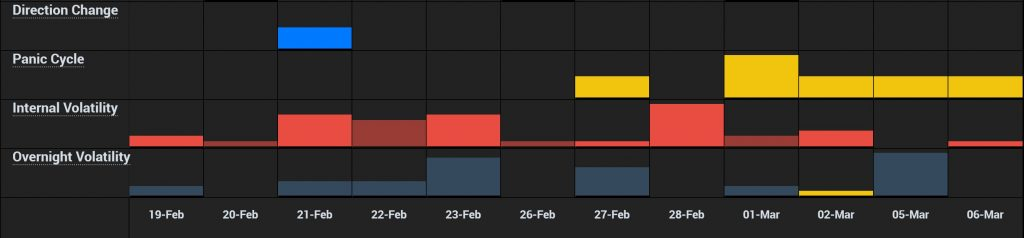# Volatility – What is It?QUESTION: Dear Martin,

In the private blog you mentioned a few times that the volatility will rise again in the week of the 12th. When you mention volatility, do you mean volatility as measured by the VIX index?

So far the VIX has lost around 1/3 this week so I suppose you mean something else?

Thanks!

JWD

ANSWER: The VIX is not a true indicator of volatility. We have three main volatility measurements and each is different.

(1) you have the traditional measurement of close to close. That is interesting, but it does not truly capture the concept of volatility.

(2) Then there is intraday volatility which we measure and simply the percentage movement between the high and low of that session. You can have a 1,000 point swing in the Dow intraday yet close nearly unchanged. The first volatility measurement would never even show a blip.

(3) The third measurement is overnight volatility. This is measured from the previous close to the open of the current day session. For example, Monday, February 5th the Dow opened at 25337.87 compared to Friday’s closing of 25520.96 gapping down.

Our indicators are intended for trading, unlike the VIX. In our Arrays, you will see Overnight Volatility, Intraday Volatility, and Panic Cycles, which are extreme moves in one direction or an outside reversal which exceeds the previous session high and penetrates the previous secession low.

The VIX is a convoluted formula that does not reflect trading but more of a trend lending itself to manipulations. Hence, the VIX is not very reliable. The VIX is a measure of expected volatility calculated as 100 times the square root of the expected 30-day variance (var) of the S&P 500 rate of return. The variance is annualized and VIX expresses volatility in percentage points.where var = (365/30) x Expected 30-day variance.

The 30-day variance is the sum of squared standard deviations st (“volatilities”) of the S&P 500 rate of return at every point in time t during the 30 days: